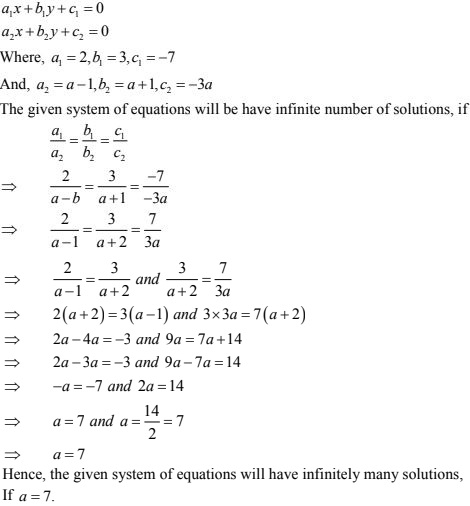# RD Sharma Solutions Chapter 3 Pair of Linear Equation in Two Variables Exercise 3.5 Class 10 Maths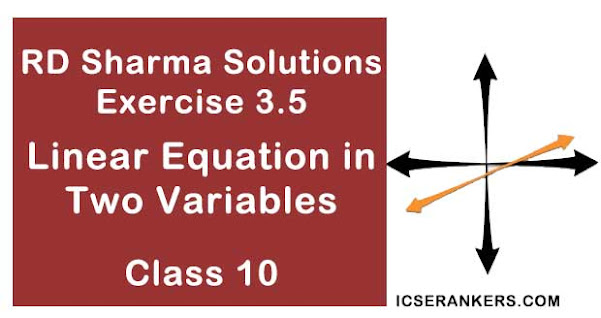Chapter Name RD Sharma Chapter 3 Pair of Linear Equation in Two Variables Book Name RD Sharma Mathematics for Class 10 Other Exercises Exercise 3.1Exercise 3.2Exercise 3.3Exercise 3.4Exercise 3.6 Related Study NCERT Solutions for Class 10 Maths

### Exercise 3.5 Solutions

In each of the following systems of equations determine whether the system has a unique solution , no solution or infinitely many solutions. In case there is a unique solution, find it : (1, -4)

1. x - 3y - 3 = 0
3x - 9y - 2 = 0

Solution

The given system of equations may be written as
x - 3y - 3 = 0
3x - 9y - 2 = 0
The given system of equations is of the form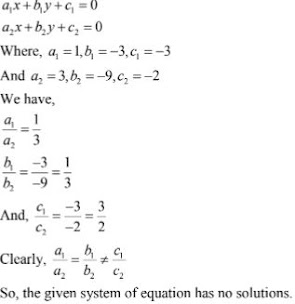2. 2x + y - 5 = 0
4x + 2y - 10 = 0

Solution

The given system of equation may be written as
2x + y - 5 =0
4x + 2y - 10 = 0
The given system of equations is of the form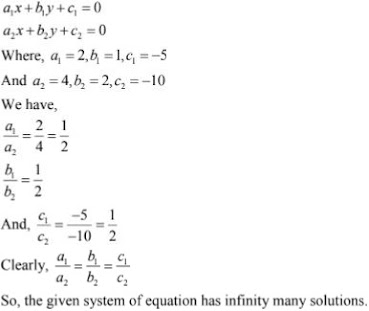3. 3x - 5y = 20
6x - 10y = 40

Solution

3x - 5y = 20
6x - 10y = 40
Compare it with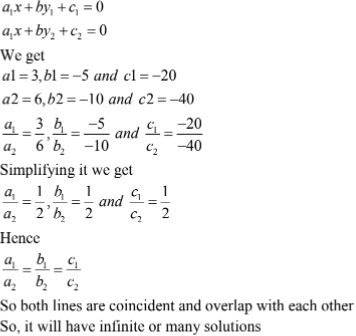4.  x - 2y - 8 = 0
5x - 10y - 10 = 0

Solution

The given system of equation may be written as
x - 2y - 8 = 0
5x - 10y - 10 = 0
The given system if equation is of the form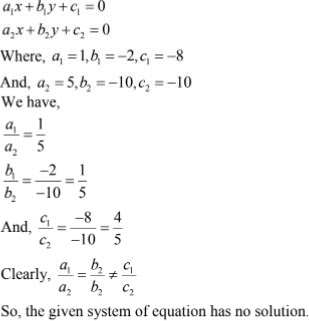5. kx + 2y - 5= 0
3x + y - 1 = 0

Solution

The given system of equation is
kx + 2y - 5= 0
3x + y - 1 = 0
The system of equation is of the form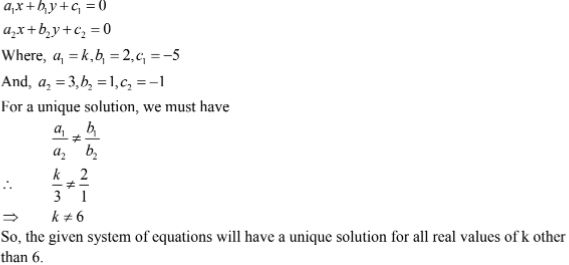6. 4x +5y + 8 = 0
2x + 2y+2 = 0
Solution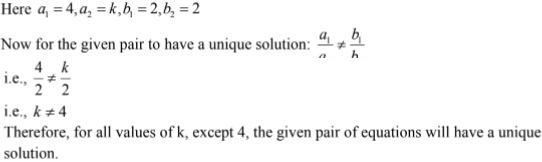7.  4x - 5y = k
2x - 3y = 12
Solution

The given system of equation is
4x - 5y - k = 0
2x - 3y - 12 = 0
The system of equation is of the form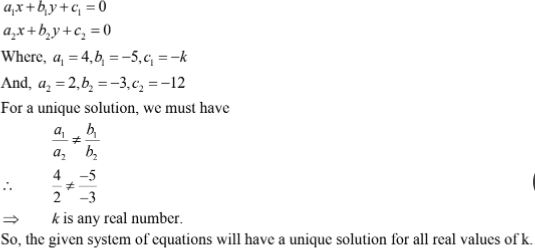8. x + 2y = 3
5x + ky + 7 = 0
Solution
The given system of equation is
x + 2y - 3 = 0
5x + ky + 7 = 0
The system of equation is of the form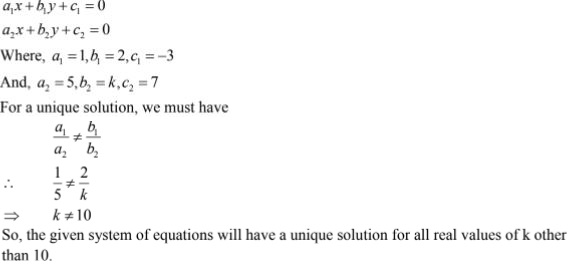Find the value of k for which each of the following systems of equations have definitely many solution : (9 - 19)

9. 2x + 3y - 5 = 0
6x - ky - 15 = 0

Solution

The given system of equation is
2x + 3y - 5 = 0
6x - ky - 15 = 0
The system of equation is of the form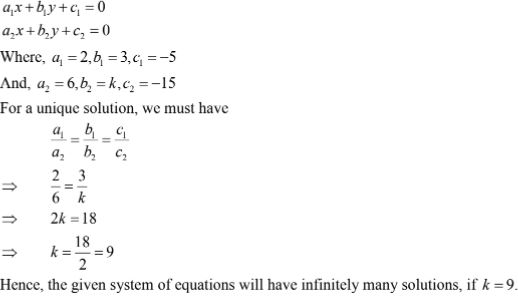10. 4x + 5y = 3
kx + 15y = 9

Solution

The given system of equation is
4x + 5y - 3 = 0
kx + 15y - 9 = 0
The system of equation is of the form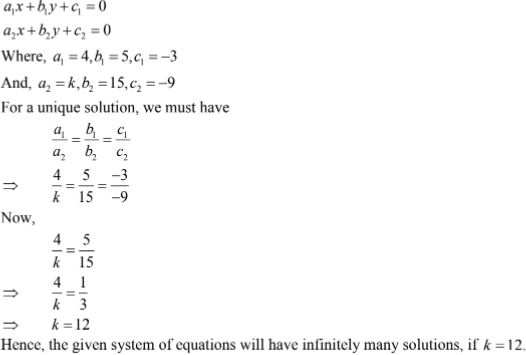11. kx - 2y + 6 = 0
4x  + 3y + 9 = 0

Solution

The given system of equation is
kx - 2y + 6 = 0
4x + 3y + 9 = 0
The system of equation is of the form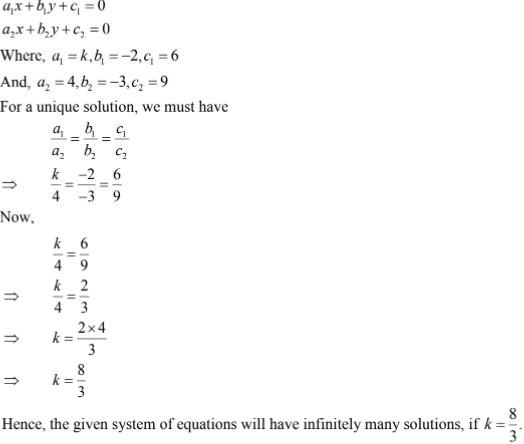12. 8x + 5y = 9
kx + 10y = 18

Solution

The given system of equation is
8x + 5y - 9  = 0
kx + 10y - 18 = 0
The system of equation is of the form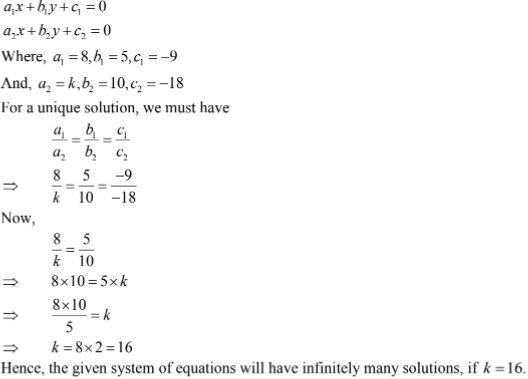13. 2x - 3y = 7
(k + 2)x - (2k + 1)y - 3(2k - 1)
Solution

The given system of equation may be written as
2x - 3y - 7 = 0
(k + 2)x - (2k + 1)y - 3(2k - 1) = 0
The system of equation is of the form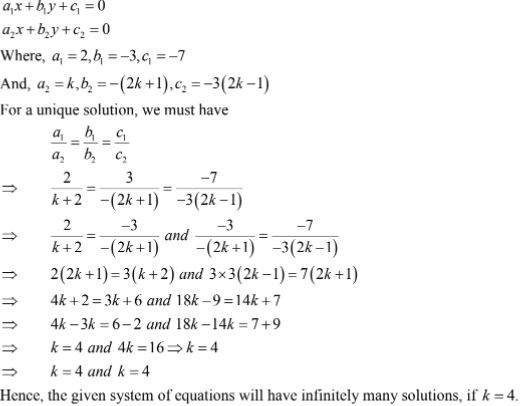14. 2x + 3y = 2
(k + 2)x + (2k + 1)y - 2(k - 1)
Solution
The given system of equation may be written as
2x + 3y - 2 = 0
(k + 2)x + (2k + 1)y - 2(k - 1) = 0
The system of equation is of the form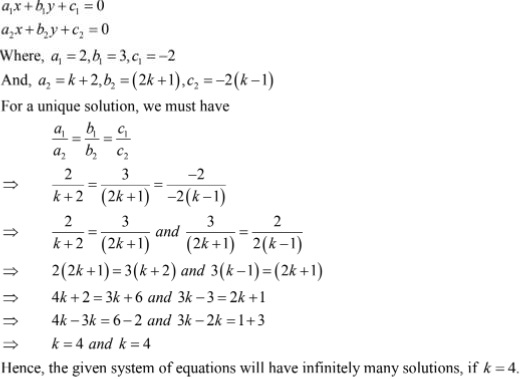15.  x + (k + 1)y = 4
(k + 1)x + 9y - (5k + 2)
Solution

The given system of equation may be written as
x + (k + 1)y - 4 = 0
(k + 1)x + 9y - (5k + 2) = 0
The system of equation is of the form16. kx + 3y - 2k + 1
2(k + 1)x + 9y - (7k + 1)
Solution

The given system of equation may be written as
kx + 3y - (2k + 1) = 0
2(k + 1)x + 9y - (7k + 1) = 0
The system of equation is of the form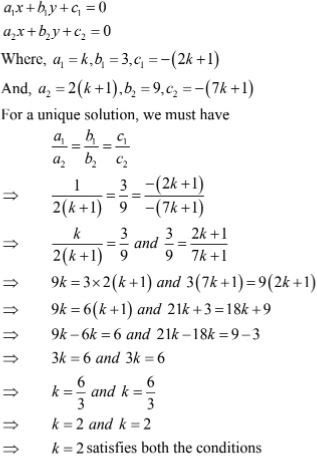Hence,  the given system of equations will have infinitely many solutions, if k = 2.

17. 2x + (k - 2)y = k
6x + (2k - 1)y - (2k + 5)
Solution

The given system of equation may be written as
2x + (k - 2)y - k = 0
6x + (2k - 1)y - (2k + 5) = 0
The system of equation is of the form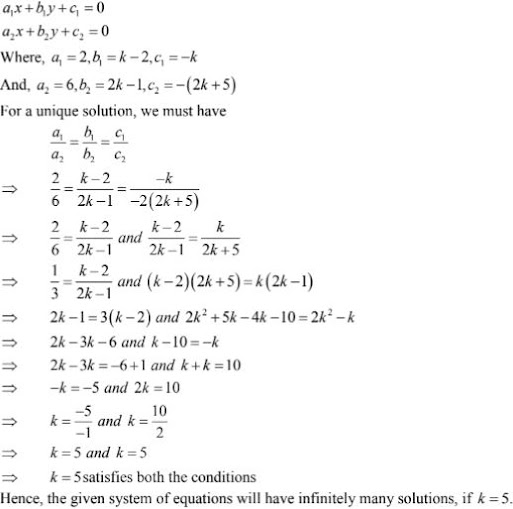18. 2x + 3y = 7
(k + 1)x + (2k - 1)y - (4k + 1)

Solution

The given system of equation may be written as
2x + 3y - 7  = 0
(k + 1)x + (2k - 1)y - (4k + 1) = 0
The system of equation is of the form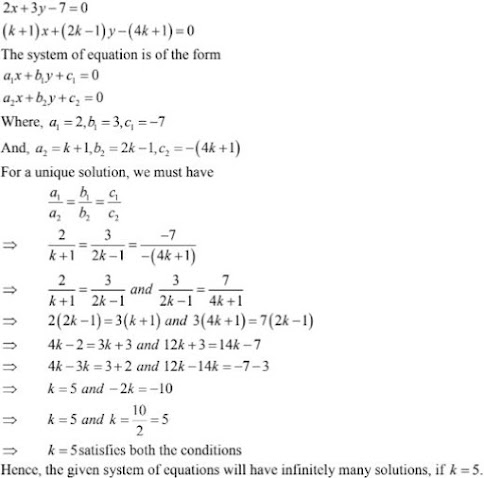19. 2x +3y = k
(k - 1)x + (k + 2)y - 3k
Solution

The given system of equation may be written as
2x + 3y - k = 0
(k - 1)x + (k + 2)y - 3k = 0
The system of equation is of the form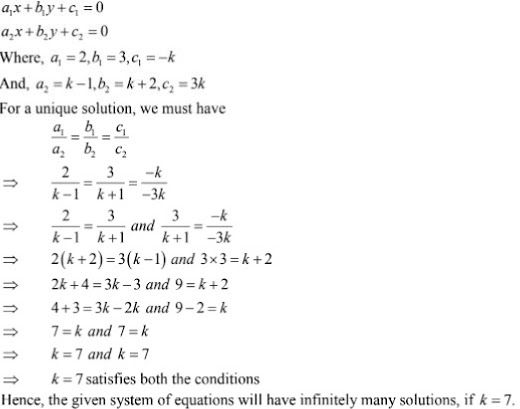Find the value of k for which the following system of equations has no solultion: (20 - 25)

20. kx - 5y = 2
6x + 2y = 7
Solution
Given,
kx - 5y = 2
6x + 2y = 7
condition for system of equations having no solution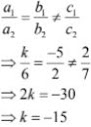21. x + 2y = 0
2x + ky - 5 = 0
Solution
The given system of equation may be written as

x + 2y = 0
2x + ky - 5 = 0
The system of equation is of the form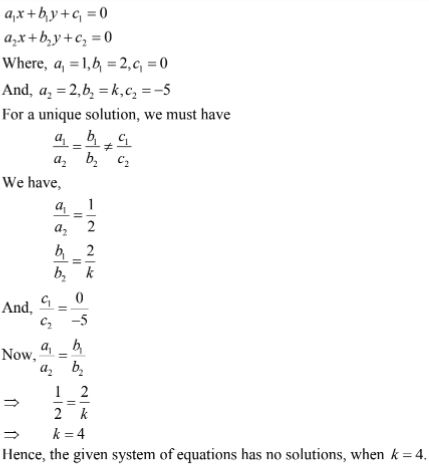22. 3x - 4y + 7 = 0
kx  + 3y - 5 = 0
Solution
The given system of equation may be written as

3x - 4y + 7 = 0
kx  + 3y - 5 = 0
The system of equation is of the form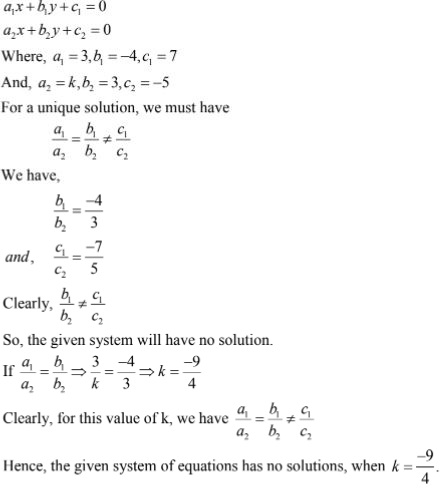23.  2x - ky +3 = 0
3x + 2y - 1 = 0
Solution
The given system of equation may be written as

2x - ky +3  = 0
3x + 2y - 1 = 0
The system of equation is of the form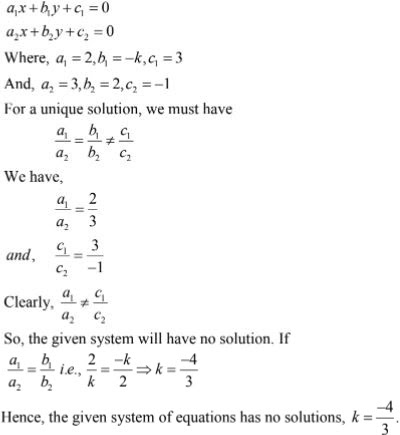24. 2x + ky - 11 = 0
5x - 7y - 5 = 0

Solution
The given system of equation is
2x + ky - 11 = 0
5x - 7y - 5 = 0
The system of equation is of the form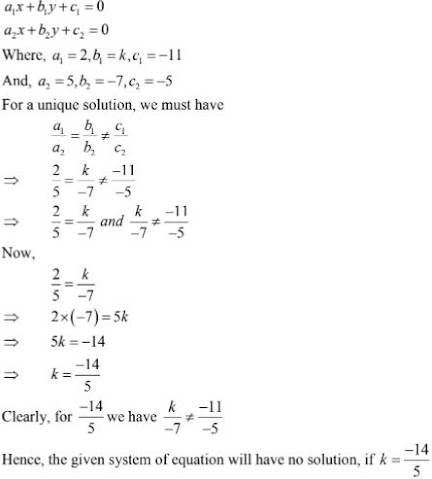25. kx + 3y = 3
12x + ky = 6
Solution

kx + 3y = 3
12x + ky = 6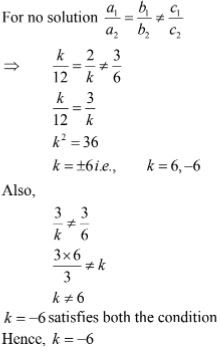26. For what value of α, the following system of equations will be inconsistent?
4x + 6y - 11 = 0
2x + ky - 7 = 0
Solution
The given system of equation may be written as
4x + 6y - 11 = 0
2x + ky - 7 = 0

The system of equation is of the form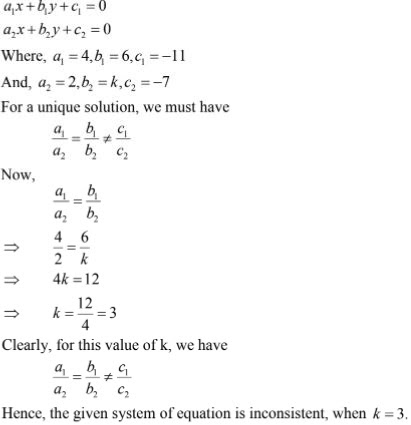27. For what value of α, the system of equation
αx + 3y = α - 3
12x + αy = α
will have no solution ?
Solution
The given system of equation may be written as
αx + 3y - (α - 3) = 0
12x + αy - α  = 0
The system of equation is of the form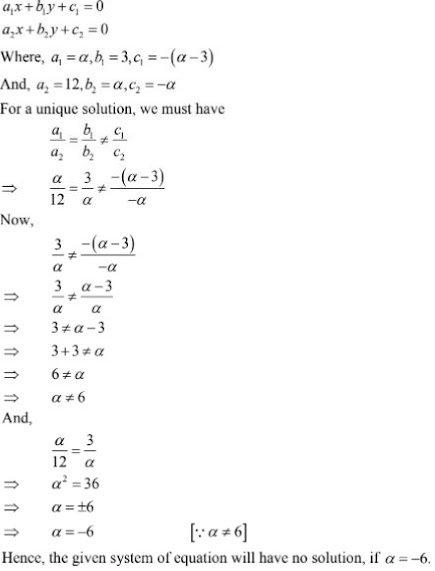28. Find the value of k for which the system
kx + 2y = 5
3x + y = 1
has (i) a unique solution, and (ii) no solution.

Solution

The given system of equation may be written as
kx + 2y - 5 = 0
3x +y - 1 = 0
It is of the form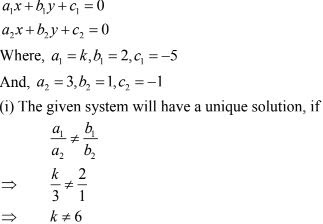So, the given system of equations will have a unique solution , if k ≠ 6

(ii) The given system will have no solution, if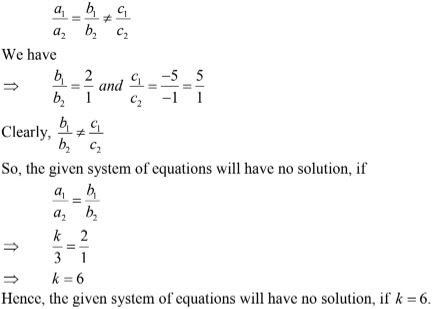29. Prove that there is a value of c (≠0) for which the system
6x + 3y = c - 3
12x + cy = c
has infinitely many solutions. Find this value.

Solution

The given system of equation may be written as
6x + 3y - (c - 3)= 0
12x + cy - c = 0
This is of the form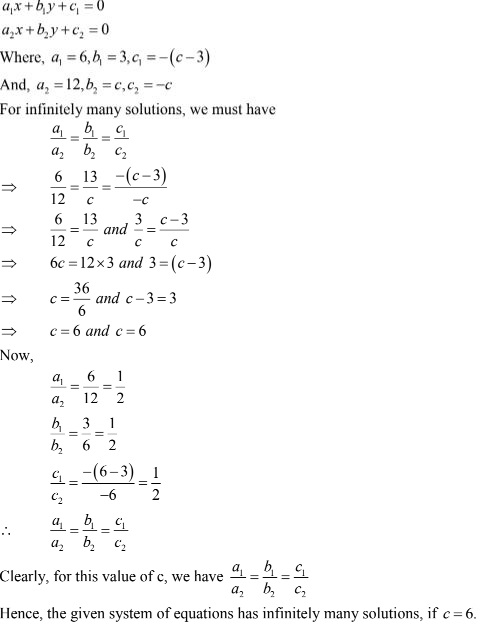30. Find the values of k for which the system
2x + ky = 1
3x - 5y = 7
will have (i) a unique solution, and (ii) no solution . Is there a value of k for which the system has infinitely many solutions ?
Solution

The given system of  equation may be written as
2x + ky - 1 = 0
3x - 5y - 7 = 0
It is of the form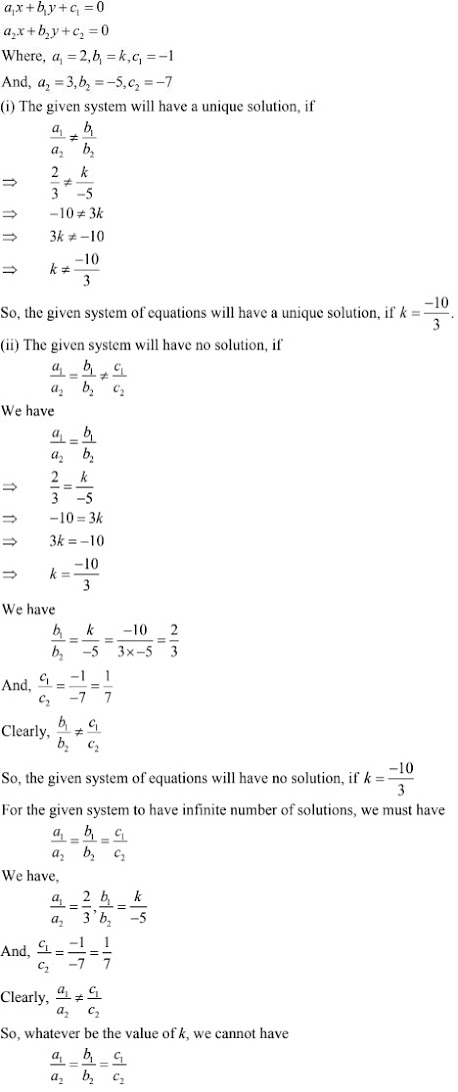31. For what value of k, the following system of equations will represent the coincident lines ?
x + 2y + 7 = 0
2x + ky + 14 = 0
Solution

The given system of equations may be written as
x + 2y + 7 = 0
2x + ky + 14 = 0

The given system of equations is of the form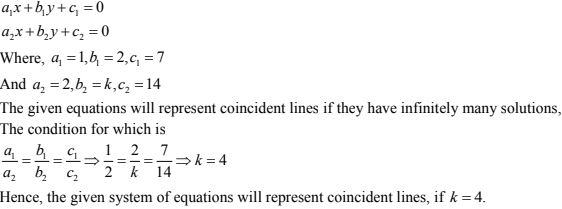32.  Obtain the condition for the following system  of linear equations to have a unique solution
ax + by = c
lx + my = n
Solution

The given system of equations may be written as

ax + by - c = 0
lx + my - n = 0
It is of the form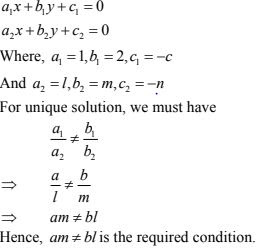33. Determine the values of a and b so that the following system of linear equations have infinitely many solutions:
(2a - 1)x + 3y - 5 = 0
3x + (b-1)y - 2 = 0
Solution

The given system of equations may be written as
(2a - 1)x + 3y - 5= 0
3x +(b-1)y - 2 = 0
It is of the form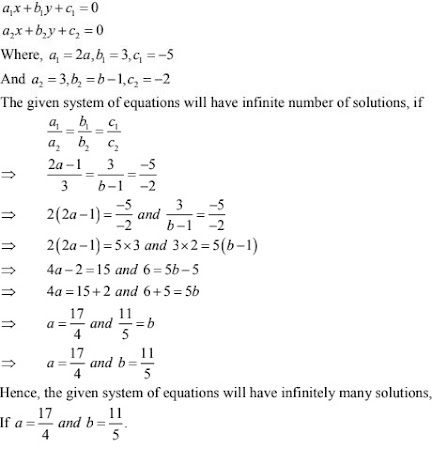34. Find the values of a and b for which the following system of linear equations has infinite number of solutions :
2x - 3y = 7
(a+b)x - (a+ b - 3)y = 4a + b
Solution

The given system of equations may be written as
2x - 3y -7 = 0
(a+b)x - (a+ b - 3)y - (4a + b) = 0
It is of the form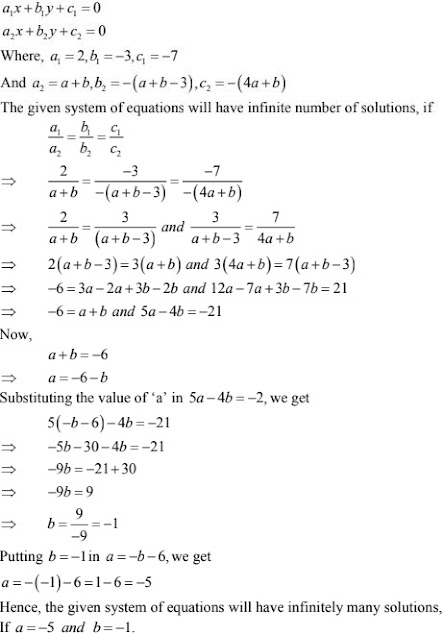35. Find the values of p and q for which the following system of linear equation has infinite number of solutions:
2x - 3y = 9
(p + q)x + (2p - q)y = 3(p + q + 1)

Solution

The given system of equations may be written as
2x - 3y - 9 = 0
(p + q)x + (2p - q)y - 3(p + q + 1) = 0
It is of the form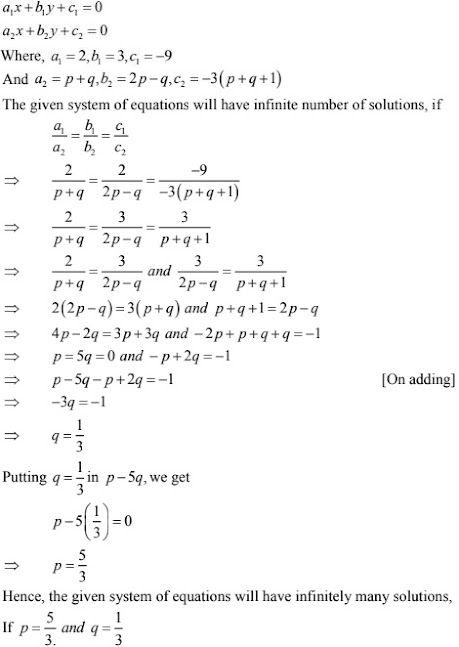36. Find the values of a and b for which the following system of equations has infinitely many solutions :
2x + 3y = 7
(a-b)x + (a+ b)y = 3a + b - 2
Solution
2x + 3y - 7 = 0
(a-b)x + (a+ b)y - (3a + b - 2 ) = 0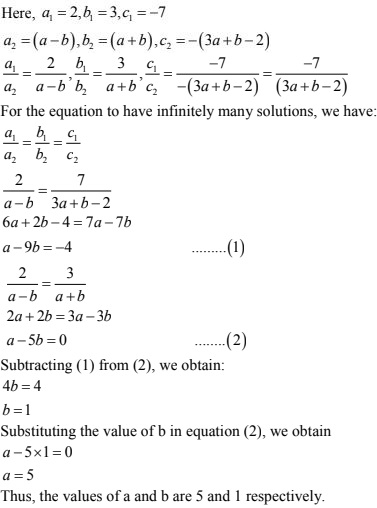(i) (2a - 1)x - 3y = 5
3x + (b - 2)y = 3
Solution

The given system of equations is

(2a - 1)x - 3y - 5 = 0
3x + (b - 2)y - 3 = 0
It is of the form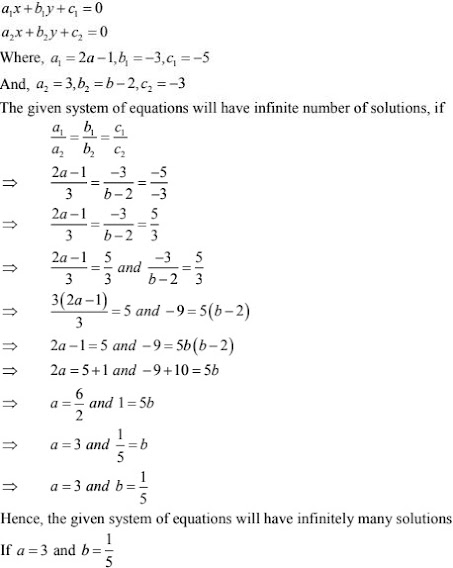(ii) 2x - (2a + 5)y = 5
(2b + 1)x - 9y = 15
Solution

The given system of equations is

2x - (2a + 5)y - 5 = 0
(2b + 1)x - 9y - 15 = 0
It is of the form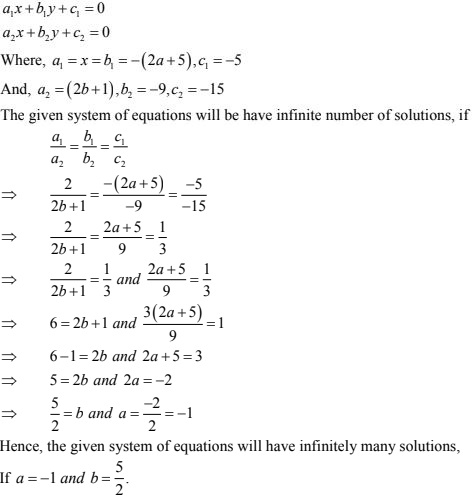(iii) (a -)x + 3y = 2
6x +(1 +2b)y = 6
Solution

The given system of equations is
(a -)x + 3y - 2 = 0
6x +(1 +2b)y  - 6  = 0
It is of the form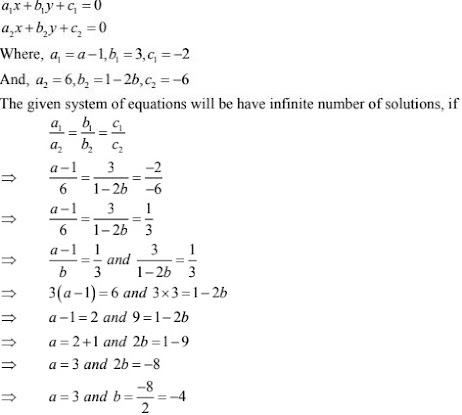Hence, the given system of equations will have infinitely many solutions,
If a = 3 and b = -4.

(iv) 3x + 4y = 12
(a +b)x + 2(a -b)y = (5a -1)
Solution

The given system of equations is
3x + 4y - 12
(a +b)x + 2(a -b)y - (5a -1) = 0
It is of the form

(v) 2x + 3y = 7
(a - 1)x + (a + 1)y = (3a - 1)
Solution

The given system of equations is
2x + 3y - 7 = 0
(a - 1)x + (a + 1)y - (3a - 1) = 0
It is of the form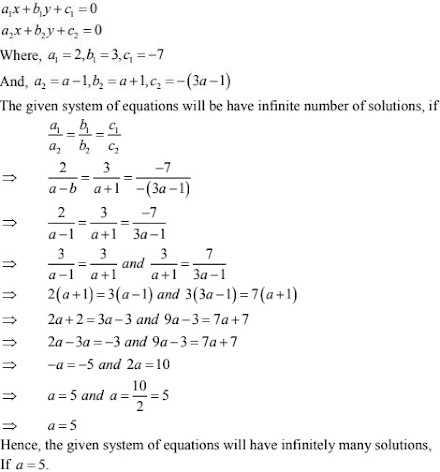(vi) 2x + 3y = 7
(a-1)x + (a+ 2)y = 3a
Solution

The given system of equations is
2x + 3y - 7 = 0
(a-1)x + (a+ 2)y - 3a  = 0
It is of the form